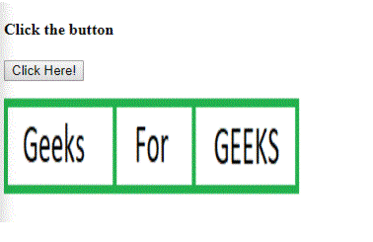# HTML | DOM Map Object

The Map Object in HTML DOM is used to create and access the <map> element. The Map Object create the portion of image clickable and accessible.
Syntax:

• It is used to return Map object.
`var x = document.getElementById("myMap");`
• It is used to create Map Object.
`var x = document.createElement("MAP");`

Property Values:

• name: It is used to set or return the value of the name attribute of an image-map.

Collection Values:

• areas: It is used to return the collection of all <area> elements in an image-map.
• images: It is used to returns the collection of all <img> and <object> elements associated with the image-map

Example 1: This example describes the getElementById() method to access the <map> element.

## html

 `` `<``html``>`   `<``head``>` `    ``<``title``> ` `        ``HTML DOM Map Object  ` `    ``` ``   `<``body``>` `    `  `    ``<``h4``>Click the button` `    `  `    ``<``button` `onclick` `= ``"GFG()"``>` `        ``Click Here!` `    ``` `    `  `    `      `<``p``>`       `    `  `    ``<``map` `id` `= ``"Geeks"` `name` `= ``"Geeks"``>` `        `  `        ``<``area` `shape`  `= ``"rect"` `coords` `= ``"0, 0, 110, 100"` `        ``alt` `= ``"Geeks"` `href` `= ` `"https://media.geeksforgeeks.org/wp-content/uploads/a1-21.png"``>`   `        ``<``area` `shape` `= ``"rect"` `coords` `= ``"110, 0, 190, 100"` `        ``alt` `= ``"For"` `href` `=` `"https://media.geeksforgeeks.org/wp-content/uploads/a1-22.png"``>`   `        ``<``area` `shape` `= ``"rect"` `coords` `= ``"190, 0, 300, 100"` `        ``alt` `= ``"GEEKS"` `href` `=` `"https://media.geeksforgeeks.org/wp-content/uploads/a1-24.png"``>` `    ``` `    `  `    ``<``img` `src` `=` `"https://media.geeksforgeeks.org/wp-content/uploads/a1-25.png"` `    ``width` `= ``"300"` `height` `= ``"100"` `alt``=``"GFG"` `usemap` `= ``"#Geeks"``>` `    `  `    ``<``p` `id` `= ``"GEEK!"``>`       `    `  `    ``<``script``>` `        ``function GFG() {` `            ``var x = document.getElementById("Geeks").areas.length;` `            ``document.getElementById("GEEK!").innerHTML = x;` `        ``}` `    ``` `                    `

Output:Example 2: This example describes the document.createElement() method to create <map> element.

## html

 `` `<``html``>` `    `  `<``head``>` `    ``<``title``> ` `        ``HTML DOM Map Object ` `    ``` `` `    `  `<``body``>` `    ``<``h4``>Click the button` `    `  `    ``<``button` `onclick` `= ``"GFG()"``>` `        ``Click Here!` `    ``` `    `  `    `      `<``p``>`       `    `  `    ``<``img` `src` `= ` `"https://media.geeksforgeeks.org/wp-content/uploads/a1-25.png"` `    ``width` `= ``"300"` `height` `= ``"100"` `usemap` `= ``"#myMap"``>` `    `  `    ``<``p` `id` `= ``"GEEK!"``>`       `    `  `    ``` `    ``<``script``>` `        ``function GFG() {` `            ``var x = document.createElement("MAP");` `            ``x.setAttribute("id", "myMap");` `            ``x.setAttribute("name", "myMap");` `            ``document.body.appendChild(x);`   `            ``var y = document.createElement("AREA");` `            ``y.setAttribute("href", ` `"https://media.geeksforgeeks.org/wp-content/uploads/a1-24.png");` `            ``y.setAttribute("shape", "rect");` `            ``y.setAttribute("coords", "190, 0, 300, 100");` `            ``document.getElementById("myMap").appendChild(y);`   `            ``document.getElementById("GEEK!").innerHTML = ` `            ``"Click on the GEEKS area in the image.";` `        ``}     ` `    ``` ``   `                    `

Output:Supported Browsers: The browser supported by DOM Map Object property are listed below: